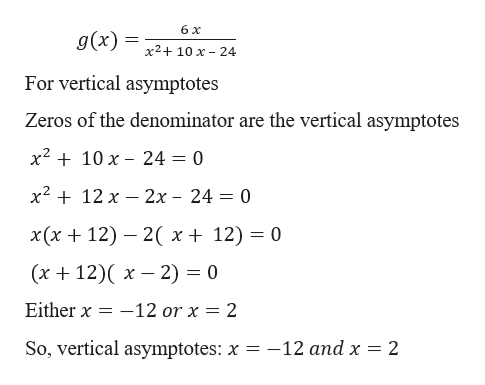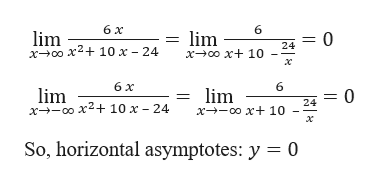Find the horizontal and vertical asymptotes of the graph of the function. (You need not sketch the graph. If an answer does not exist, enter DNE.)g(x) = (6 x)/(x^2 + 10 x - 24)

Question

Find the horizontal and vertical asymptotes of the graph of the function. (You need not sketch the graph. If an answer does not exist, enter DNE.)
g(x) = (6 x)/(x^2 + 10 x - 24)

Step 1

Given,help_outlineImage Transcriptionclose6 x g(x) х2+ 10 х - 24 For vertical asymptotes Zeros of the denominator are the vertical asymptotes x210 x 24 = 0 х2 + 12 х — 2х - 24 — 0 х(х + 12) — 2( х+ 12) — 0 (х + 12)( х — 2) %3D 0 -12 or x 2 Either x So, vertical asymptotes: x = -12 and x = 2 fullscreen
Step 2

For horizontal a...help_outlineImage Transcriptionclose6х 6 lim x00 x210 x - 24 lim x0o x 10 = 0 24 х 6х 6 lim x00 x2+10 x - 24 lim = 0 24 х+-0о х+ 10 x So, horizontal asymptotes: y = 0 fullscreen

Want to see the full answer?

See Solution

Want to see this answer and more?

Our solutions are written by experts, many with advanced degrees, and available 24/7

See Solution
Tagged in

Calculus